challenging practice

Write the missing numbers.

Question 1.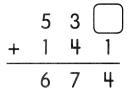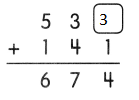Explanation:
In ones place 3 and 1 makes 4
so, missing number is 3

Question 2.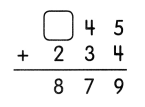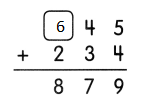Explanation:
In hundreds place 6 hundreds and 2 hundreds make 8 hundreds

Question 3.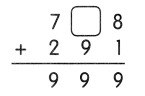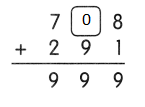Explanation:
In tens place 0 tens  + 9 tens  is 9 tens

Question 4.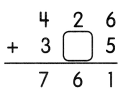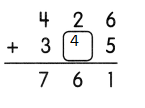Explanation:
In tens place 2 tens and 4 tens make 6 tens

Question 5.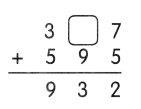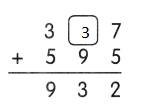Explanation:
7 ones + 5 ones = 12
1 tens and 2 ones
In tens place 3 tens 9 tens and 1 ten = 13 tens

Question 6.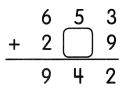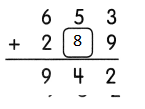Explanation:
In ones place 3 ones and 9 ones make 12 ones
That is 1 tens and 2 ones
1 ten will be carry
So, 8 ten +5 ten + 1 ten ten = 8 + 6 = 14 tens

Do these.

Question 7.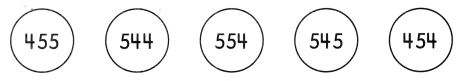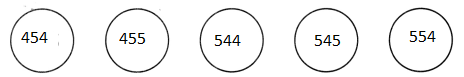Explanation:
The numbers are written in least to greatest

a. Order the numbers from greatest to least.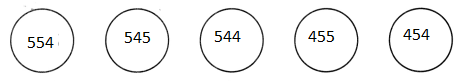Explanation:
The number are written in Decending order

Explanation:
The least number in the given numbers is 454 + 100 = 554

problem Solving

Make two 3-digit numbers from the numbers below. Use each number only once. What are the two 3-digit numbers that give the greatest answer when you add them?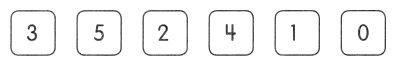____ ____ _____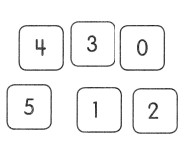Explanation:
5, 1, 2 = 5 + 1 + 2 = 8
and 4, 3, 0 = 4 + 3 + 0 = 7
are the two 3-digit numbers that give the greatest answer when you add them

Chapter Review/Test

Vocabulary

Fill in the blanks with words from the box.
The words may be used more than once.

Question 1.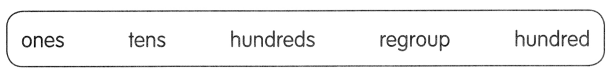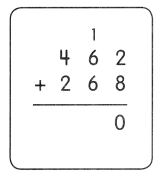Step 1
2 ones + 8 ones = 10 ones
__________ the ones.
10 ones = 1 ten 0 ones

Explanation:
2 ones + 8 ones = 10 ones
Regroup the ones.
10 ones = 1 ten 0 ones
Step 2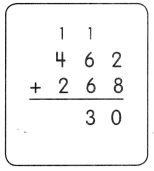1 ten + 6 tens + 6 tens = 13 tens
___________ the tens.
13 tens = 1 _________ 3 ____

Explanation:
1 ten + 6 tens + 6 tens = 13 tens
Regroup the tens.
13 tens = 1tens 3 ones
Step 3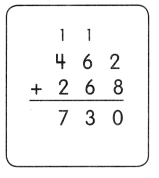1 hundred + 4 hundreds + 2 hundreds
= 7 hundreds
462 + 268 = 730

Explanation:
1 hundred + 4 hundreds + 2 hundreds
= 7 hundreds
462 + 268 = 730

Concepts and Skills

Then match the problems with the same answer.

Question 2.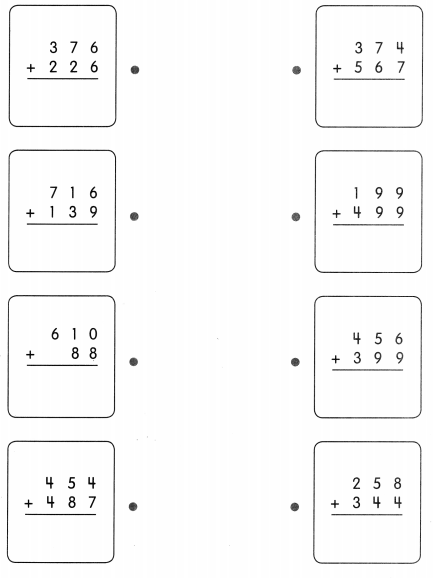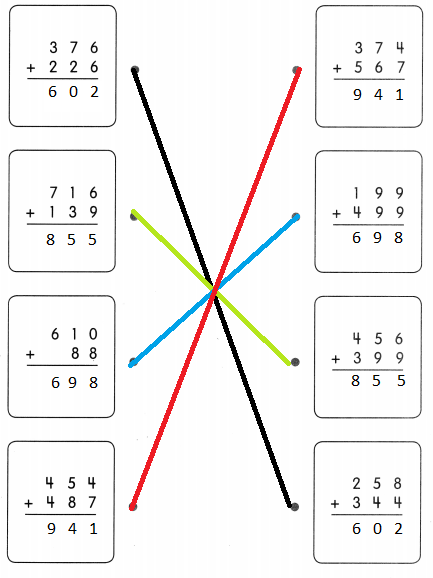Explanation:
The addition of three digits number is found on both sides
And matched the sum of numbers

Problem Solving

Solve.

Question 3.
Mr. Thomas drives 173 miles on Monday. On Tuesday, he drives 216 miles. How many miles does he drive in all?
He drives ___ miles in all.
He drives ___ miles in all.
Explanation:
Mr. Thomas drives 173 miles on Monday.
On Tuesday, he drives 216 miles.
173 + 216 = 389 miles does he drive in all

Question 4.
A carpenter has 362 pieces of lumber. He needs another 228 pieces of lumber to build a bridge. How many pieces of lumber does he need to build the bridge?
He needs ___ pieces of lumber to build the bridge.
He needs ___ pieces of lumber to build the bridge
Explanation:
A carpenter has 362 pieces of lumber.
He needs another 228 pieces of lumber to build a bridge.
362 + 228 = 590 pieces of lumber does he need to build the bridge

Solve.

Question 5.
A movie theater sells 294- tickets to the first show. It sells 457 tickets to the second show. How many tickets does it sell in all?
It sells ___ tickets in all.
It sells ___ tickets in all
Explanation:
A movie theater sells 294- tickets to the first show.
It sells 457 tickets to the second show.
294 + 457 = 751 tickets does it sell in all

Question 6.
Shantel has 546 stickers in her collection. She has 278 fewer stickers than Sherice. How many stickers does Sherice have in her collection?
Shantel has ___ stickers in her collection.